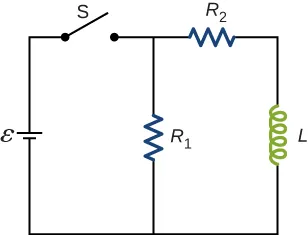University Physics Volume 2

# Conceptual Questions

University Physics Volume 2Conceptual Questions

### 14.1Mutual Inductance

1.

Show that $NΦm/INΦm/I$ and $ε/(dI/dt),ε/(dI/dt),$ which are both expressions for self-inductance, have the same units.

2.

A 10-H inductor carries a current of 20 A. Describe how a 50-V emf can be induced across it.

3.

The ignition circuit of an automobile is powered by a 12-V battery. How are we able to generate large voltages with this power source?

4.

When the current through a large inductor is interrupted with a switch, an arc appears across the open terminals of the switch. Explain.

### 14.2Self-Inductance and Inductors

5.

Does self-inductance depend on the value of the magnetic flux? Does it depend on the current through the wire? Correlate your answers with the equation $NΦm=LI.NΦm=LI.$

6.

Would the self-inductance of a 1.0 m long, tightly wound solenoid differ from the self-inductance per meter of an infinite, but otherwise identical, solenoid?

7.

Discuss how you might determine the self-inductance per unit length of a long, straight wire.

8.

The self-inductance of a coil is zero if there is no current passing through the windings. True or false?

9.

How does the self-inductance per unit length near the center of a solenoid (away from the ends) compare with its value near the end of the solenoid?

### 14.3Energy in a Magnetic Field

10.

Show that $LI2/2LI2/2$ has units of energy.

### 14.4RL Circuits

11.

Use Lenz’s law to explain why the initial current in the RL circuit of Figure 14.12(b) is zero.

12.

When the current in the RL circuit of Figure 14.12(b) reaches its final value $ε/R,ε/R,$ what is the voltage across the inductor? Across the resistor?

13.

Does the time required for the current in an RL circuit to reach any fraction of its steady-state value depend on the emf of the battery?

14.

An inductor is connected across the terminals of a battery. Does the current that eventually flows through the inductor depend on the internal resistance of the battery? Does the time required for the current to reach its final value depend on this resistance?

15.

At what time is the voltage across the inductor of the RL circuit of Figure 14.12(b) a maximum?

16.

In the simple RL circuit of Figure 14.12(b), can the emf induced across the inductor ever be greater than the emf of the battery used to produce the current?

17.

If the emf of the battery of Figure 14.12(b) is reduced by a factor of 2, by how much does the steady-state energy stored in the magnetic field of the inductor change?

18.

A steady current flows through a circuit with a large inductive time constant. When a switch in the circuit is opened, a large spark occurs across the terminals of the switch. Explain.

19.

Describe how the currents through $R1andR2R1andR2$ shown below vary with time after switch S is closed.20.

Discuss possible practical applications of RL circuits.

### 14.5Oscillations in an LC Circuit

21.

Do Kirchhoff’s rules apply to circuits that contain inductors and capacitors?

22.

Can a circuit element have both capacitance and inductance?

23.

In an LC circuit, what determines the frequency and the amplitude of the energy oscillations in either the inductor or capacitor?

### 14.6RLC Series Circuits

24.

When a wire is connected between the two ends of a solenoid, the resulting circuit can oscillate like an RLC circuit. Describe what causes the capacitance in this circuit.

25.

Describe what effect the resistance of the connecting wires has on an oscillating LC circuit.

26.

Suppose you wanted to design an LC circuit with a frequency of 0.01 Hz. What problems might you encounter?

27.

A radio receiver uses an RLC circuit to pick out particular frequencies to listen to in your house or car without hearing other unwanted frequencies. How would someone design such a circuit?

Order a print copy

As an Amazon Associate we earn from qualifying purchases.